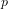Hostname: page-component-797576ffbb-lm8cj Total loading time: 0 Render date: 2023-12-08T21:29:47.883Z Has data issue: false Feature Flags: { "corePageComponentGetUserInfoFromSharedSession": true, "coreDisableEcommerce": false, "useRatesEcommerce": true } hasContentIssue false

# Coleman integration for even-degree models of hyperelliptic curves

Published online by Cambridge University Press:  01 April 2015

## Abstract

Core share and HTML view are not possible as this article does not have html content. However, as you have access to this content, a full PDF is available via the ‘Save PDF’ action button.

The Coleman integral is a$p$-adic line integral that encapsulates various quantities of number theoretic interest. Building on the work of Harrison [J. Symbolic Comput. 47 (2012) no. 1, 89–101], we extend the Coleman integration algorithms in Balakrishnan et al. [Algorithmic number theory, Lecture Notes in Computer Science 6197 (Springer, 2010) 16–31] and Balakrishnan [ANTS-X: Proceedings of the Tenth Algorithmic Number Theory Symposium, Open Book Series 1 (Mathematical Sciences Publishers, 2013) 41–61] to even-degree models of hyperelliptic curves. We illustrate our methods with numerical examples computed in Sage.

Type
Research Article
Information

## References

Balakrishnan, J. S., ‘Iterated Coleman integration for hyperelliptic curves’, ANTS-X: Proceedings of the Tenth Algorithmic Number Theory Symposium , Open Book Series 1 (eds Howe, E. W. and Kedlaya, K. S.; Mathematical Sciences Publishers, 2013) 4161.Google Scholar
Balakrishnan, J. S., Besser, A. and Müller, J. S., ‘Quadratic Chabauty:$p$ -adic height pairings and integral points on hyperelliptic curves’, J. reine angew. Math., to appear.Google Scholar
Balakrishnan, J. S., Bradshaw, R. W. and Kedlaya, K. S., ‘Explicit Coleman integration for hyperelliptic curves’, Algorithmic number theory , Lecture Notes in Computer Science 6197 (eds Hanrot, G., Morain, F. and Thomé, E.; Springer, 2010) 1631.Google Scholar
Chabauty, C., ‘Sur les points rationnels des courbes algébriques de genre supérieur à l’unité’, C. R. Acad. Sci. Paris 212 (1941) 882885.Google Scholar
Coleman, R. F., ‘Dilogarithms, regulators and p-adic L-functions’, Invent. Math. 69 (1982) no. 2, 171208.Google Scholar
Coleman, R. F., ‘Effective Chabauty’, Duke Math. J. 52 (1985) no. 3, 765770.Google Scholar
Coleman, R. F., ‘Torsion points on curves and p-adic abelian integrals’, Ann. of Math. (2) 121 (1985) no. 1, 111168.Google Scholar
Coleman, R. F. and de Shalit, E., ‘ p-adic regulators on curves and special values of p-adic L-functions’, Invent. Math. 93 (1988) no. 2, 239266.Google Scholar
Flynn, E. V., ‘Descent via isogeny in dimension 2’, Acta Arith. 66 (1994) no. 1, 2343.Google Scholar
Flynn, E. V., ‘A flexible method for applying Chabauty’s theorem’, Compos. Math. 105 (1997) no. 1, 7994.Google Scholar
Harrison, M. C., ‘An extension of Kedlaya’s algorithm for hyperelliptic curves’, J. Symbolic Comput. 47 (2012) no. 1, 89101.Google Scholar
Kedlaya, K. S., ‘Counting points on hyperelliptic curves using Monsky–Washnitzer cohomology’, J. Ramanujan Math. Soc. 16 (2001) 323338; erratum J. Ramanujan Math. Soc. 18 (2003) 417–418.Google Scholar
Stein, W. A. et al. , ‘Sage mathematics software, version 6.2’ (The Sage Development Team, 2014) http://www.sagemath.org.Google Scholar